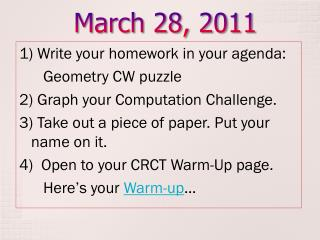DownloadDownload PresentationMarch 28, 2011

# March 28, 2011

Télécharger la présentation## March 28, 2011

- - - - - - - - - - - - - - - - - - - - - - - - - - - E N D - - - - - - - - - - - - - - - - - - - - - - - - - - -
##### Presentation Transcript

1. March 28, 2011 1) Write your homework in your agenda: Geometry CW puzzle 2) Graph your Computation Challenge. 3) Take out a piece of paper. Put your name on it. 4) Open to your CRCT Warm-Up page. Here’s your Warm-up…

2. CRCT Geometry Review Let’s see what you can do

3. Constructions • The word bisect means to cut into two congruent halves • What is the difference between a line, a line segment and a ray? • Line extends in two directions without end • Line segment is part of a line that has two endpoints • Rayis part of a line that has one end point

4. Constructions Copying an angle Constructing parallel lines Bisecting a line segment or Constructing a perpendicular bisector Constructing perpendicular lines Copying a line segment Bisecting an angle

5. Constructions: What Comes Next? NOW YOU TRY: Joel wants to bisect angle <FGH. What comes first? To bisect PQ, Maria began by swinging equal arcs from P and Q above the line segment and labeling the intersection X. What should she do next? Use a straightedge to draw a ray. Swing equal arcs from P and Q below the line segment.

6. Constructions: What Comes Next? NOW YOU TRY: Uri wants to draw a line through point P that is parallel to KD. What is the first step Uri should take? Use a straightedge to draw a ray through point P that crosses KD. A line segment has a length of 36 cm. If Lisa bisects the segment, what is the length of each segment? 18cm

7. Transformations A sofa is pushed across the floor of a living room. Which type of transformation is this? The hour hand of a clock from 7 to 8. Which type of transformation is this? You look at yourself in the mirror. Which type of transformation is this new image? Translation Rotation Reflection

8. Transformations K’ (1,-7) K’ (3,4) K’ (-4,-3)

9. Transformations NOW YOU TRY: Start: (-6,9) Reflect over y-axis Finish: Start: (-4,-2) Translate (-3,10) Finish: Start: (-9,0) Rotate 270o counterclockwise Finish: (6,9) (-7,8) (0,9)

10. y y B’ B 3 x x 3 C’ –3 C Transformations NOW YOU TRY: Reflect triangle ABC over the y-axis. What are the new coordinates? Translate triangle ABC (-5,-3) What are the new coordinates? (0,0), (-2,3), (-2,-3) (-4,2), (0,2), (-4,-2)

11. Dilations Original x Scale Factor = Dilation SF < 1 is a reduction SF > 1 is an enlargement If a percent of dilation is given, multiply by the decimal version of the percent 4 x SF = 5.6 SF = 1.4 4 5.6

12. Dilations NOW YOU TRY: Dilate (9,6) by a scale factor of 1/3 What is the scale factor for N(6,-2) and its dilation of N’(15, -5)? A square in a coordinate plane undergoes a 40% dilation. One corner of the square originally had coordinates (4,6). What are the dilated coordinates? (3,2) SF = 5/2 (1.6, 2.4)

13. Similar Figures The word corresponding means same position in similar figures The word congruentmeans exactly the same size and shape The word similarmeans same shape, but different size. Sides are proportionate. Scale factor = length ratio= perimeter ratio Scale factor2 = area ratio

14. Similar Figures NOW YOU TRY: A rectangle has an area of 30 feet. A similar rectangle has an area of 270 feet. What is the scale factor? What is the length of the missing side? 3 t = 150 cm

15. Similar Figures NOW YOU TRY: What is the scale factor, length ratio and area ratio? 14 km x 12 km 6 km Scale Factor = ½, length ratio = ½, area ratio = ¼

16. Forming 3D Figures Rotations and translations of two dimensional figures can be used to form 3D figures. • A circle rotating about a line through its center makes a sphere • A triangle rotating about a vertical line makes a cone • A square that has been translated makes a rectangular prism • A circle that has been translated makes a cylinder

17. Cross Sections Cross sections are views inside a 3D object after it has been sliced • Slices can be made horizontally, vertically, or diagonally Cross Sections of a Cylinder: • Horizontal: circle • Vertical: rectangle • Diagonally: oval

18. Forming 3D Figures and Cross Sections NOW YOU TRY: What 3D figure will be formed if rectangle ABCD is rotated about AB? What 3D figure is formed when you translate a circle? A rectangular prism is cut vertically, what is the cross section? Suppose a cone is cut by a plane. Which of the following cross sections is NOT possible? Circle Ellipse Square Triangle cylinder cylinder square square